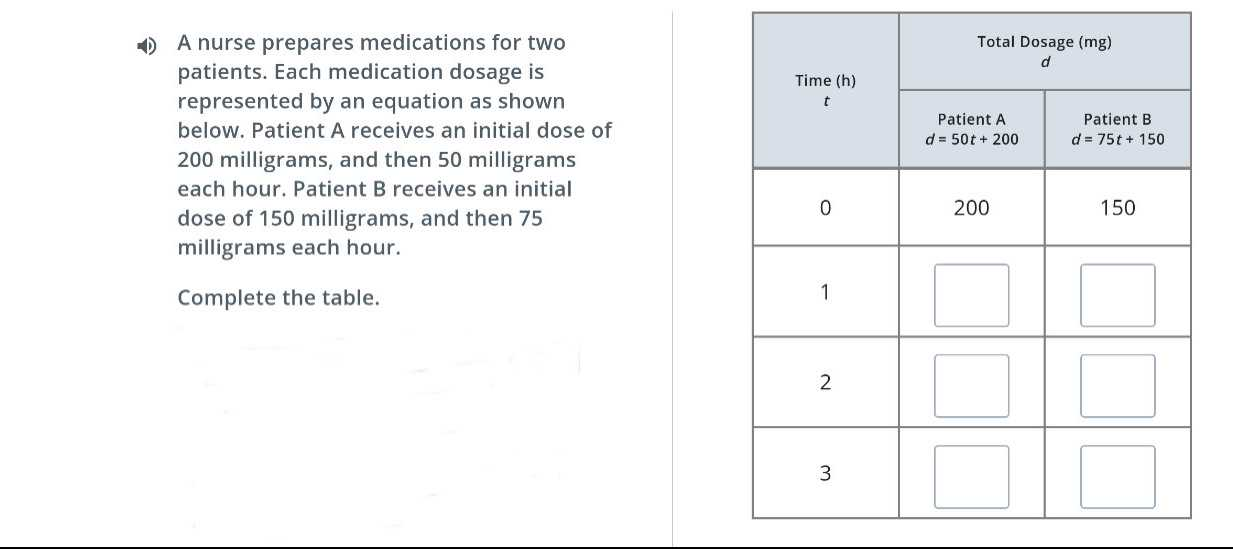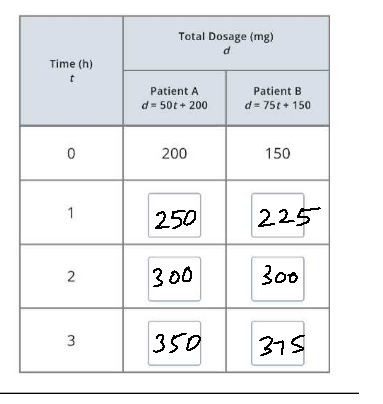### ¿Todavía tienes preguntas de matemáticas?

Pregunte a nuestros tutores expertos
Algebra
Pregunta1) A nurse prepares medications for two patients. Each medication dosage is represented by an equation as shown below. Patient A receives an initial dose of $$200$$ milligrams, and then $$50$$ milligrams each hour. Patient B receives an initial dose of $$150$$ milligrams, and then $$75$$ milligrams each hour. Complete the table.